The Menger Sponge Fractal

The Menger sponge is a popular fractal that generalizes Cantor sets and Sierpinski triangles. Its fractal dimension is between two and three.

Contents

Karl Menger

Karl Menger (1902-1985) was a 20th-century Austrian-American mathematician. Most of his career was at the Illinois Institute of Technology in Chicago. He made contributions to geometry and game theory. But he is best known for his cube-like fractal known as a "sponge".

The Sponge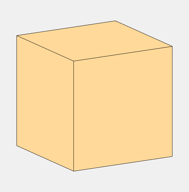Trisect the big cube into 3x3x3 = 27 smaller cubes, like a Rubik's cube without the labels. Remove the center cube from each of the six faces and the cube at the very center. That leaves these 27 - 7 = 20 smaller cubes at level one.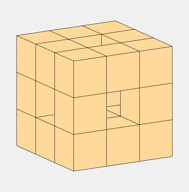Repeat the process on each of these 20 cubes to generate 20x20 = 400 even smaller cubes at level two.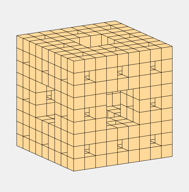Once more. This is level three with 800 tiny cubes and lots of holes.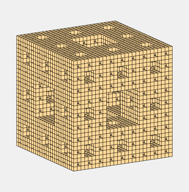With the resolution available on our computer screens, or on a printed page, there is little to be gained by going beyond level three. Each cube occupies only a few pixels and the level four cubes would be 20 times smaller.

And there are 20 times as many of them. That's 160,000 subpixel sized cubes. Even though I wasn't going to be able to view it, I did complete one computation at level four. It requiries more main memory than the 32 gigabytes of RAM that I have on my laptop. It had to use virtual storage and ran for almost 15 minutes.

Two- or three-dimensional?

The solid volume is reduced by a factor of 20/27 at each level. So if you theoretically keep going past level three or four, the volume approaches zero. On the other hand, the surface area becomes infinite. The result is a fractal that is somewhere between two- and three-dimensional. In 1918, Felix Hausdorff introduced fractal dimension. It turns out that the Menger sponge has Hausdorff dimension log(20)/log(3), which is about 2.727.

Light on the subject.

So far, we've been working in the dark. Let's shed some light on the subject. The big cube we started with is actually (a simulation of) solid gold.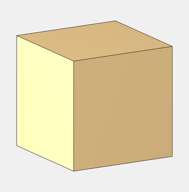The light strikes various surfaces at different angles to produce different shades of gold. We can now easily see the holes.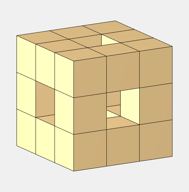At level two we have carved out almost half of the gold.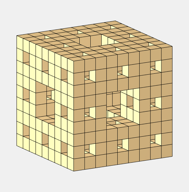With the light and shading, level three takes about five seconds to compute and plot. It is somewhat darker than the other plots because the cubes edges are beginning to dominate.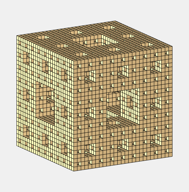Tilt

If you tilt the sponge very slightly, you can see that the holes are channels passing all the way through.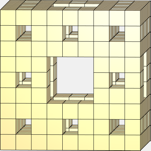Animation

I try to have animated .gifs in all of my blog posts.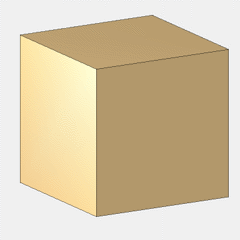menger

Here is my code. The function menger gets things started. There are two input parameters. The first specifies the desired level of refinement. A single solid cube is level = 0. Increasing level by one multiplies the number of cubes, and consequently both the execution time and the memory required, by a factor of 20. Any level greater than 3 is impractical.

The second input parameter is true or false. True turns on a camlight so you can see more detail. With false there is no lighting. Lighting takes more time, especially if you expect to manipulate the sponge after it has been computed.

The 8-by-3 array V provides the vertices of a cube of half-width 3. Starting with this width means that many of the subsequent computations are done with no roundoff error. This is important when the size of the cubes approaches the graphics resolution.

function menger(level,lit)
% Fractal cube, the Menger sponge.
% menger(level) generates 20^level cubes.
% menger(level,true) is illuminated.
% menger(level,false) is not illuminated, so it is faster.
V = [-3 -3 -3; -3 -3 3; -3 3 -3; -3 3 3;
3 -3 -3;  3 -3 3;  3 3 -3;  3 3 3];
init_fig(lit)
sponge(V,level)
end

sponge

This is where all the action takes place. The function sponge is recursive. It calls itself repeatedly, passing arrays v representing the vertices of small cubes. The v arrays are obtained from the original V by scaling with inverse powers of 3 and translating with vectors [x y z] made from all possible combinations of -2, +2 and 0. A typical v is

[-3 -3 -3; -3 -3 3; -3 3 -3; -3 3 3
3 -3 -3;  3 -3 3;  3 3 -3;  3 3 3]/9  +  [0 -2  2]

The vectors [x y z] are coordinates of cube centers. The addition of a vector to an array is a case of MATLAB's singleton expansion. The translated v may not be nearby, but it is somewhere in the sponge. And all of the cubes in the sponge are reached by the recursion.

Now a key point. The function nnz in the innermost loop counts the number of nonzero components in a vector. The cubes that would be obtained by translation with nnz([x y z]) equal to 0 or 1 are skipped because they are precisely the cubes that provide the holes in the fractal.

function sponge(v,level,lit)
if level > 0
v = v/3;
for x = [-2 0 2]
for y = [-2 0 2]
for z = [-2 0 2]
if nnz([x y z]) > 1
sponge(v+[x y z],level-1,lit)
end
end
end
end
else
cube(v,lit)
end
end

cube

This function uses patch to plot the six faces of a gold cube. If lighting is required some specular reflectance parameters are set by material.

function cube(v,lit)
f = [ 1 5 7 3
3 7 8 4
1 3 4 2
2 4 8 6
1 2 6 5
5 6 8 7];
gold = [1 .85 .60];
p = patch(Vertices = v, ...
Faces = f, ...
FaceColor = gold, ...
LineWidth = 0.5);
if lit
golden = [0.7,0.5,0.3,1.0,0.2];
material(p,golden)
end
end

init_fig

Initializes the figure, the axis and, if desired, a light pointed at the cube from the viewing position. The name windowbuttonmotionfcn is the longest identifier in MATLAB and a very handy function. We need one of these here so the light follows along when we rotate a sponge.

function init_fig(lit)
axis(4*[-1 1 -1 1 -1 1])
axis equal off vis3d
rotate3d on
view(60,15)
if lit
camlight(-45,10);
set(gcf,'windowbuttonmotionfcn',@wbmf);
end
end

wbmf

function wbmf(~,~)
a = gca;
l = findobj(a,'type','light');
camlight(l,-45,10);
end

Software

My code is available here.

The Web has lots more about the Menger sponge. People have built ones that you can walk through. People have printed them with 3D printers. Google "Menger sponge images" and click on "see all".

Wikipedia has a good article. https://en.wikipedia.org/wiki/Menger_sponge.

I particularly enjoyed this video. https://www.youtube.com/watch?v=fWsmq9E4YC0.

The New York Times even has an article. https://www.nytimes.com/2011/06/28/science/28math-menger.html.

Thanks

Thanks to Ed Angel for introducing me to Menger's creation and to Bob Blaine for some much needed help with graphics.

Published with MATLAB® R2021a

|

댓글

댓글을 남기려면 링크 를 클릭하여 MathWorks 계정에 로그인하거나 계정을 새로 만드십시오.